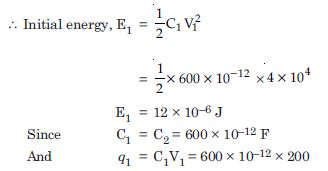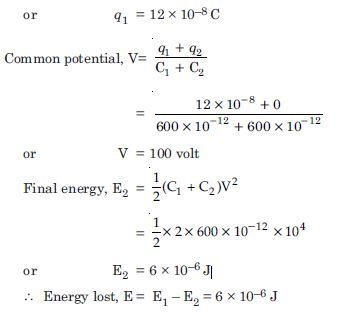# NCERT Solutions for Class 12 Physics Chapter 2 - Electrostatic Potential and Capacitance

Get free NCERT Solutions on the Aasoka platform to achieve good grades in the Class 12 board exams. The solutions are formulated by the subject matter experts in a simple and lucid language that can easily be understood by all students. The NCERT Solutions for Class 12th makes it easier for students to get a good grasp on the concepts or topics related to the Physics chapter “Electrostatic Potential and Capacitance”.

The class 12th chapter of Physics “Electrostatic Potential and Capacitance” explains electrostatic potential, problems based on the effective capacitance of capacitors, energy stored in a capacitor, important derivations and formulas, development of electrostatic potential, etc.

##### Question 1:

Two charges 5 × 10–8 C and – 3 × 10–8 C are located 16 cm apart. At what point(s) on the line joining the two charges is the electric potential zero ? Take the potential at infinity to be zero.

Given
q1 = 5×10–8 C, r = 16 cm= 0.16 m
q2 = –3 × 10–8 C
Let potential be zero at a distance x metre from
positive charge q1
$\therefore$ r1 = x metre
r2 = (0.16 – x) metre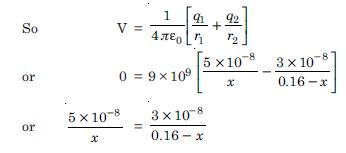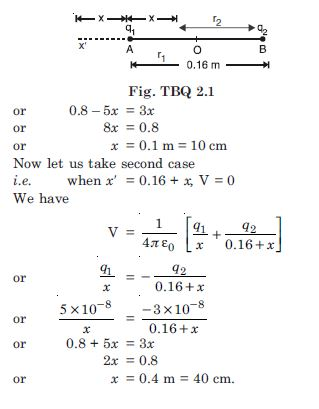##### Question 2:

A regular hexagon of side 10 cm has a charge 5 $\mathrm{\mu }$C at each of its vertices. Calculate the potential at the centre of the hexagon.

From the figure TBQ 2.2 we have OP = OQ = OR = OS = OT = OU = r = 10 cm = 0.1 m And given q = 5 $\mathrm{\mu }$C = 5 × 10–6 C
$\therefore$ Potential at O due to all the charges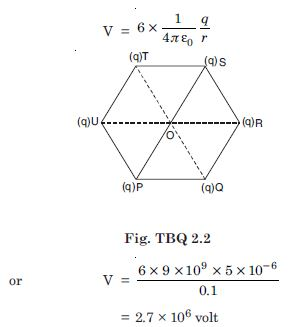##### Question 3:

Two charges 2 $\mathrm{\mu }$C and –2 $\mathrm{\mu }$C are placed at points A and B 6 cm apart. Identify an equipotential surface of the system.
What is the direction of the electric field at every point on this surface ?

The plane normal to AB and passing through its mid-point has zero potential everywhere hence the plane is equipotential.

Normal to the plane is the direction AB.

##### Question 4:

A spherical conductor of radius 12 cm has a charge of 1.6 × 10–7 C distributed uniformly on its surface. What is the electric field

1. inside the sphere
2. just outside the sphere
3. at point 18 cm from the centre of the sphere ?

(a) Zero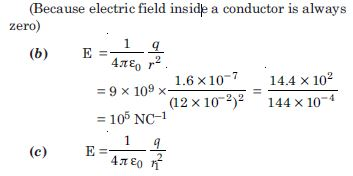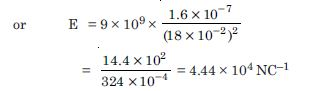##### Question 5:

A parallel plate capacitor with air between the plates has a capacitance of 8pF (1pF = 10–12 F). What will be the capacitance if the distance between the plates is reduced by half, and the space between them is filled with a substance of dielectric constant 6 ?

The capacitance of capacitor with air as dielectric is given by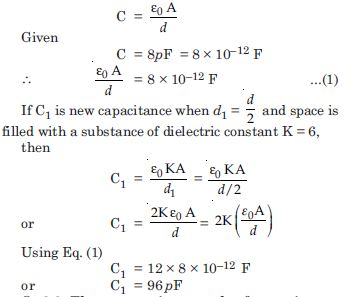##### Question 6:

Three capacitors each of capacitance 9pF are connected in series.

What is the total capacitance of the combination ?

What is the potential difference across each capacitor, if the combination is connected to a 120 volt supply ?

Given C 1= C 2= C 3 = 9 pF = 9 × 10 –12 F; V = 120 volt.
Total capacitance of the series combination is given by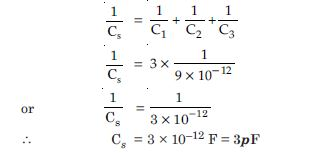Let q be charge on each capacitor. Then, sum of the potential differences across their plates must be equal to 120 V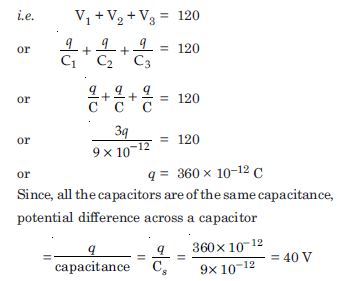##### Question 7:

Three capacitors of capacitance 2pF, 3pF and 4pF are connected in parallel.

What is the total capacitance of the combination ?

Determine the charge on each capacitor, if the combination is connected to a 100 V supply.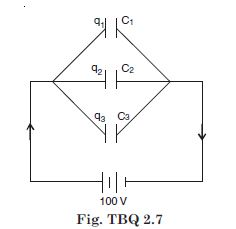Here C1 = 2pF; C2 = 3pF; C3 = 4pF ; V = 100 volt
Total capacitance of the parallel combination is given by
C = C1 + C2 + C3 = 2 + 3 + 4 = 9 pF.
Let q1, q2 and q 3 be the charges on C1, C2 and C3 respectively. In the parallel combination, the potential difference across each capacitor will be equal to the supply voltage i.e. 100 V.
Therefore, q1 = C1 V = 2 × 1012 × 100
= 2 × 10–10 C,
q2 = C2 V=3×10–12×100 =3×10–10 C and q 3 = C 3 V = 4×10–12×100=4×10 –12 C.

##### Question 8:

In a parallel plate capacitor with air between the plates, each plate has an area of 6 × 10–3 m2 and the distance between the plates is 3 mm. Calculate the capacitance if this capacitor is connected to a 100 V supply, what is the charge on each plate of the capacitor ?

Given
A = 6 × 10–3 m2, d = 3 mm = 3 × 10–3 m C0 = ?
V0 = 100 V, q = ?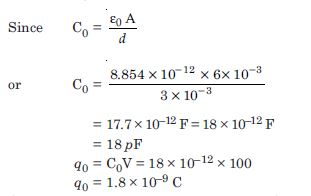##### Question 9:

Explain what would happen if in the capacitor given in exercise 2.8, a 3mm thick mica sheet (of dielectric constant = 6) were inserted between the plates.

1. While the voltage supply remained connected.
2. After the supply was disconnected.

(a) When supply voltage remains connected
V = 100 volt (voltage remains constant) Capacitance C = KC 0 = 6 × 18 = 108 pF Charge q =1.8 × 10 –9 C (remains constant)

(b) After the supply is disconnected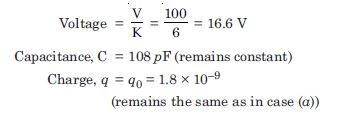##### Question 10:

A 12 pF capacitor is connected to a 50 V battery. How much electrostatic energy is stored in the capacitor ?

Given C = 12 pF = 12 × 10–12 C
V = 50 volt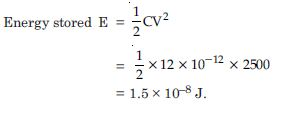##### Question 11:

A 600 pF capacitor is charged by a 200 V supply. It is then disconnected from the supply and is connected to another uncharged 600 pF capacitor. How much electrostatic energy is lost in the process ?